# # 4 数据流分析-基础

## # 4.1 重新审视迭代算法

• 给定一个具有k个结点的程序流程图CFG，对于CFG中的每个结点 $n$ ，迭代算法的每一次迭代都会更新 $OUT[n]$

• 设数据流分析的定义域 为 $V$ ，我们可以定义一个 $k$ 元组（k-tuple）来表示每次迭代后的分析值：

$(OUT[n_1], OUT[n_2], ..., OUT[n_k]) \in V \times V \times ... \times V = V^k$
• 每次迭代可以视为将 $V^k$ 中的某个值映射为 $V^k$ 中的另一个值，通过状态转移方程和控制流约束式，这个过程可以抽象为一个函数 $F_{V^k \to V^k}$

• 然后这个算法会输出一系列的k元组，直到某两个连续输出的k元组完全相同的时候算法终止。

...... ......

• 算法保证能够终止吗？也就是说不动点一定存在吗？或者说数据流分析是否总会有一个解决方案？

• 如果是的话，不动点是只有一个吗？不止一个的话，我们算法终止的不动点是最好的那个吗？

• 算法什么时候会到达不动点？或者说我们什么时候能够得到一个解决方案？

## # 4.2 唤醒一些记忆深处的数学

### # 4.2.1 偏序

1. 自反性（Reflexivity）$\forall x\in P, x \preceq x$

2. 反对称性（Antisymmetry）$\forall x, y\in P, x \preceq y \wedge y \preceq x \Rightarrow x = y$

3. 传递性（Transitivity）$\forall x, y, z \in P, x \preceq y \wedge y \preceq z \Rightarrow x \preceq z$

### # 4.2.2 格

• 如果 $\forall a, b\in P$$a\vee b$ 存在，则我们称 $(P, \preceq)$联合半格（Joint Semilattice）

• 如果 $\forall a, b\in P$$a\wedge b$ 存在，则我们称 $(P, \preceq)$交汇半格（Meet Semilattice）

• $P = P_1 \times P_2 \times ...\times P_n$
• $(x_1, x_2, ..., x_n) \preceq (y_1, y_2, ..., y_n) \Leftrightarrow (x_1 \preceq y_1) \wedge (x_2 \preceq y_2) \wedge ... \wedge (x_n \preceq y_n)$
• $(x_1, x_2, ..., x_n) \wedge (y_1, y_2, ..., y_n) = (x_1 \wedge y_1, x_2 \wedge y_2, ..., x_n \wedge y_n)$
• $(x_1, x_2, ..., x_n) \vee (y_1, y_2, ..., y_n) = (x_1 \vee y_1, x_2 \vee y_2, ..., x_n\vee y_n)$

### # 4.2.3 不动点

$f(\bot), f(f(\bot)), ..., f^{h + 1}(\bot)$

$f(\top), f(f(\top)), ..., f^{h + 1}(\top)$

$\bot \preceq f(\bot) \preceq f^2(\bot) \preceq ... \preceq f^{h + 1}(\bot)$

$\forall x, y, z\in L$ ，若 $x \preceq y$ ，由于 $x \wedge z \preceq x \preceq y$$x \wedge z \preceq z$ ，于是有 $x \wedge z \preceq y \wedge z$ ，从而 $\wedge$ 是单调的。

$\forall x, y, z \in L$ ，若$x \preceq y$，由于 $x \preceq y \preceq y \vee z$$z \preceq y \vee z$ ，于是有 $x \vee z \preceq y \vee z$ ，从而 $\vee$ 也是单调的。

### # 4.2.4 分配律

$x \preceq x \vee y$$f(x)$ 单调，有 $f(x) \preceq f(x \vee y)$ ，同理 $f(y) \preceq f(x \vee y)$ ，于是 $f(x) \vee f(y) \preceq f(x \vee y)$

$x \wedge y \preceq x$$f(x)$ 单调，有 $f(x \wedge y) \preceq f(x)$ ，同理 $f(x \wedge y) \preceq f(y)$ ，于是 $f(x \wedge y) \preceq f(x) \wedge f(y)$

## # 4.3 基于格的数据流分析框架

• $D$ （Direction）：数据流的方向——正向或者逆向；

• $L$ （Lattice）：一个包含值集 $V$ 的域（即 $V$ 的幂集）的格以及一个交汇操作符（Meet Operator）或者联合操作符（Joint Operator）；

• $F$ （Function Family）：一个从 $V$$V$ 的转移函数族（Transfer Function Family）。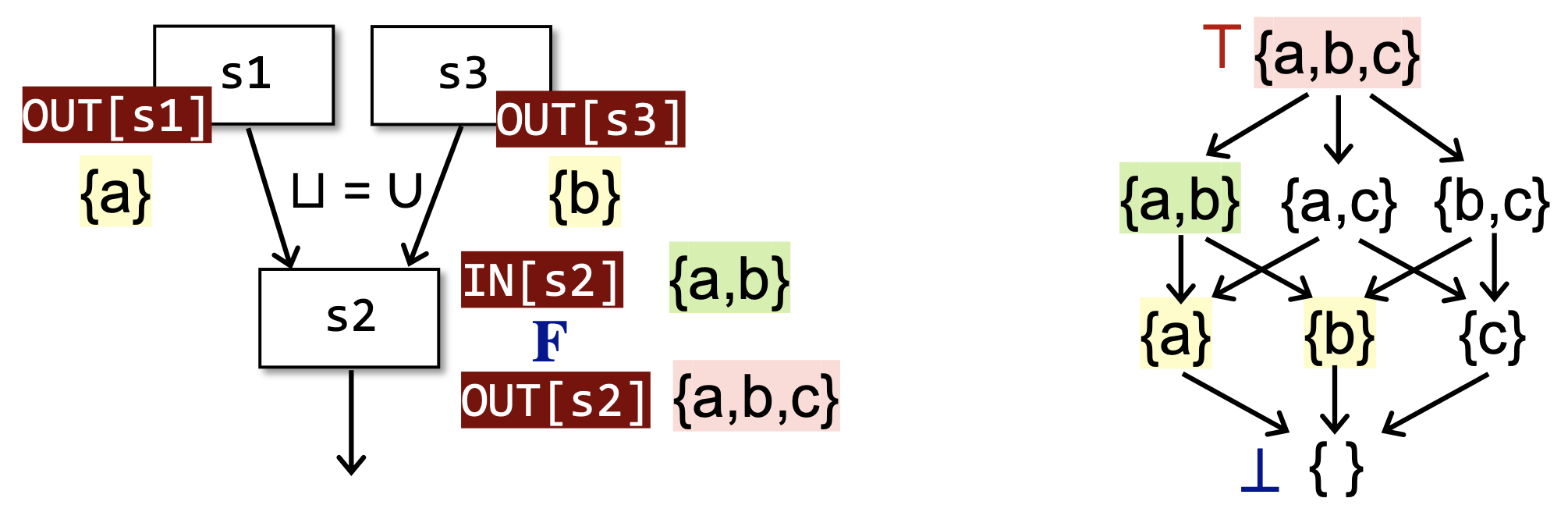$F$ 有两个部分组成，一个部分是状态转移方程 $f_{L\to L}$ ，从定理3.4后面的证明中我们可以发现，定义可达性分析的状态转移方程是单调的，类似的，变量活性分析和可用表达式分析的状态转移方程都是单调的。在我们进行其他分析的时候，我们设计状态转移方程时，需要保证其单调性。也就是说，一个设计糟糕的状态转移方程可能是不单调的，从而导致我们的迭代算法无法终止，或者无法求出符合预期的结果。

## # 4.4 格视角下的可能性分析与必然性分析

### # 4.4.1 可能性分析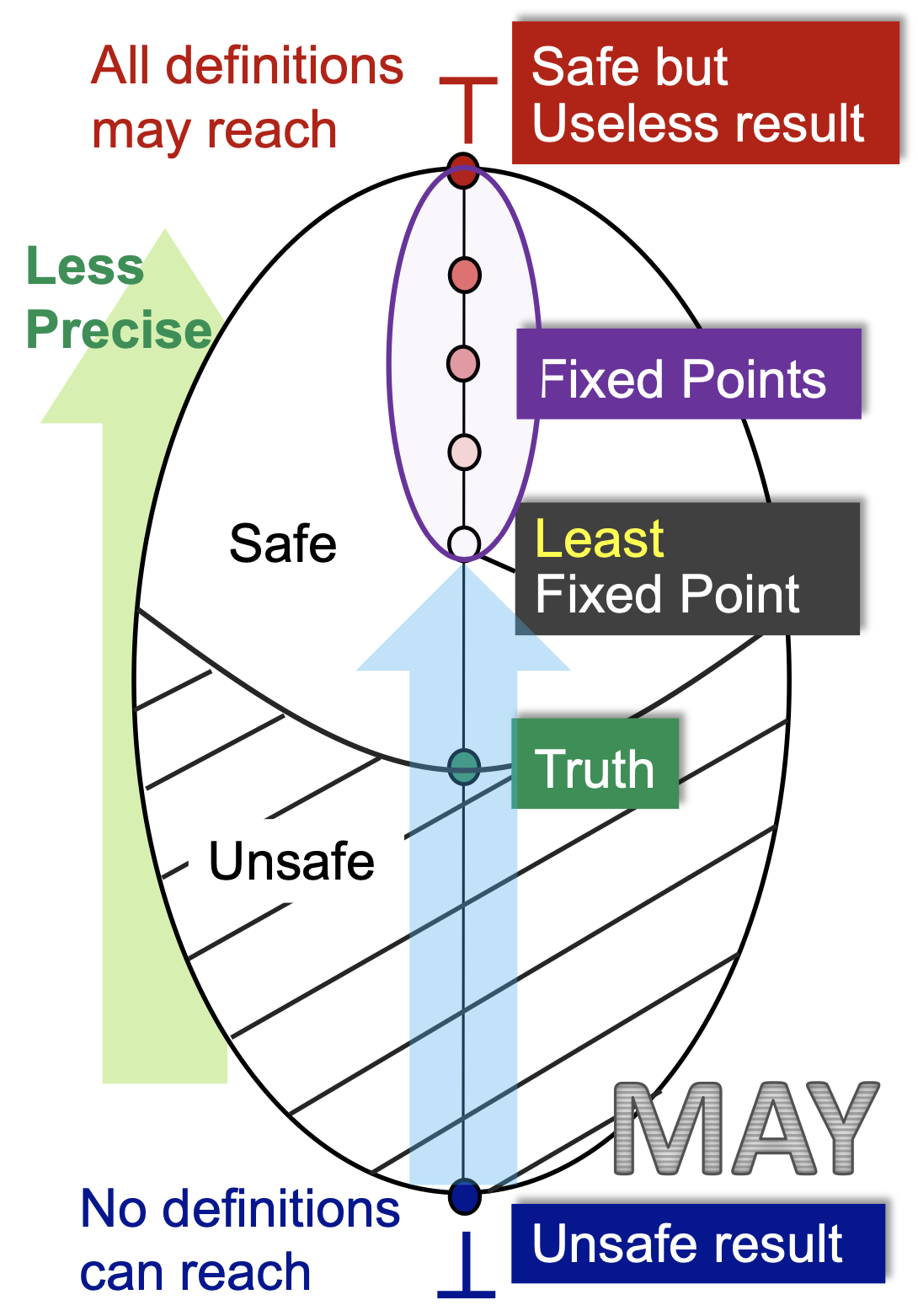### # 4.4.2 必然性分析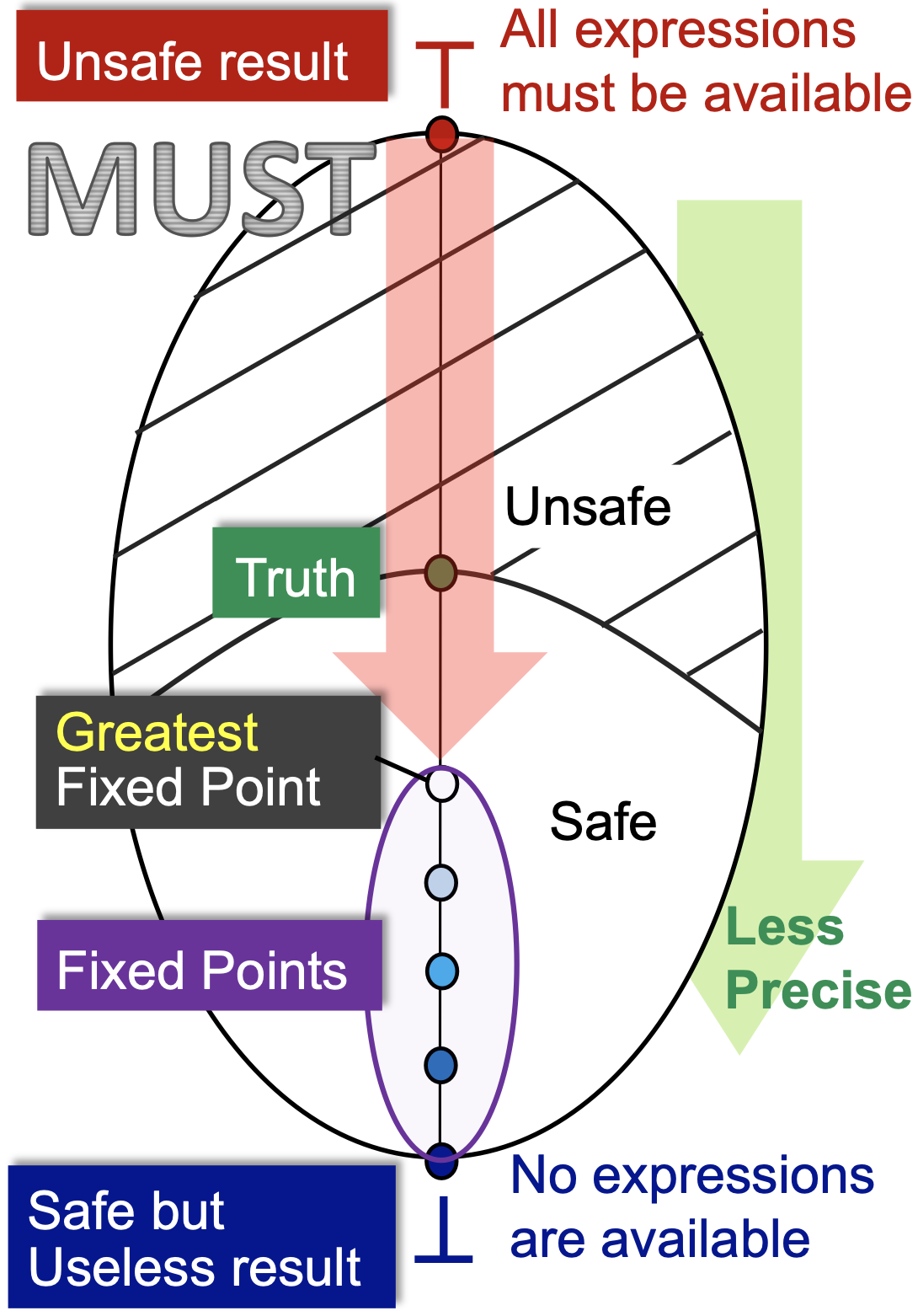## # 4.5 算法精度分析

### # 4.5.1 全路交汇的解决方案

$F_P = f_{s_i} \circ f_{s_{i - 1}} \circ ... \circ f_{s_2} \circ f_{s_1}$

• 考虑从程序入口到 $s_i$ 处的路径 $P$ 的状态转移方程 $F_P$，所有路径的集合记为 $Paths(ENTRY, s_i)$

• 使用联合或者交汇操作来求这些值的最小上界或者最大下界。

$MOP[s_i] = \bigvee_{\forall P \in Paths(ENTRY, s_i)} F_P(OUT[ENTRY])$

$MOP[s_i] = \bigwedge_{\forall P \in Paths(ENTRY, s_i)} F_P(OUT[ENTRY])$

### # 4.5.2 MOP与迭代算法的比较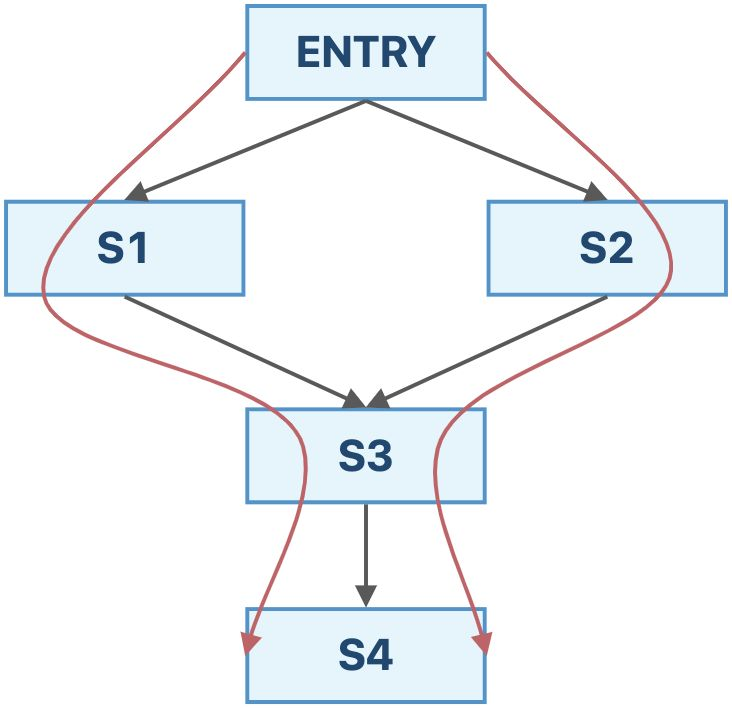$IN[s_4] = f_{s_3}(f_{s_1}(OUT[ENTRY]) \vee f_{s_2}(OUT[ENTRY]))$

$IN[s_4] = f_{s_3}(f_{s_1}(OUT[ENTRY])) \vee f_{s_3}(f_{s_2}(OUT[ENTRY]))$

## # 4.6 常量传播

### # 4.6.2 问题分析

#### # 控制流约束

• $(x, v) \wedge (x, NAC) = (x, NAC)$ ，变量和任意常量交汇还是变量，因为我们需要保证是一个常量，采用的是必然性分析的策略；

• $(x, UNDEF) \wedge (x, v) = (x, v)$ ，未初始化的变量并不是我们这个问题的关注点（言下之意出了Undefined的错误，锅由Reaching Definitions背），我们当它不存在即可。

• $(x, v_1) \wedge (x, v_2) = \begin{cases}(x, v_1),\quad if\ v_1 = v_2\\(x, NAC),\quad otherwise\end{cases}$ ，两个不同的值汇聚到某一点，说明这个变量不是常量，否则，在这个程序点处，我们可以将其视为常量。

#### # 状态转移方程

$F: OUT[s] = gen_s \cup (IN[s] - \{(x, \_)\})$

• s: x = c; //c is a constant ，则 $gen_s = \{(x, c)\}$

• s: x = y; ，则 $gen_s = \{(x, val(y))\}$

• s: x = y op z; ，则 $gen_s = \{(x, f(y, z))\}$ ，其中，

$f(y,z) = \begin{cases}val(y)\ op\ val(z)\quad if\ y\ and\ z\ are\ constants\\ NAC\quad if\ y\ or\ z\ is NAC\\ UNDEF\quad otherwise\end{cases}$

### # 4.6.3 区别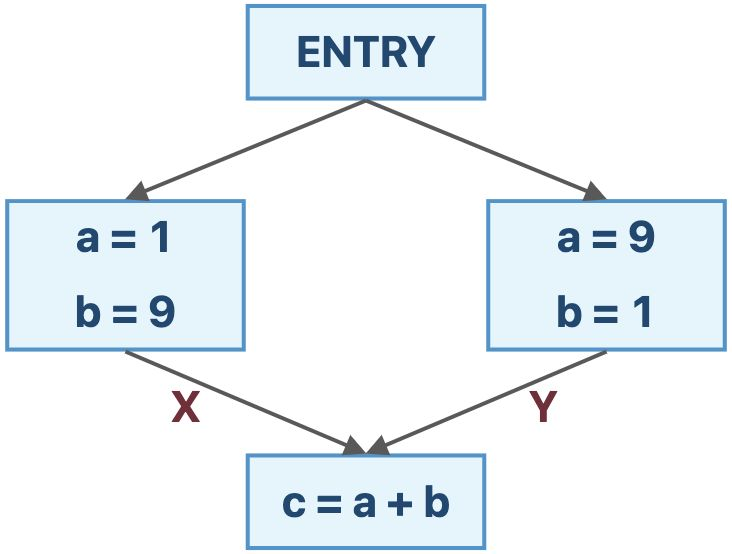$F(X \wedge Y) = \{(a, NAC), (b, NAC), (c, NAC)\}$

$F(X) \wedge F(Y) = \{(a, NAC), (b, NAC), (c, 10)\}$

## # 4.8 自检问题

1. 如何从函数的角度来看待数据流分析的迭代算法？

2. 格和全格的定义是什么？

3. 如何理解不动点定理？

4. 怎样使用格来总结可能性分析与必然性分析？

5. 迭代算法提供的解决方案与MOP相比而言精确度如何？

6. 什么是常量传播（Constant Propagation）分析？

7. 数据流分析的工作表算法（Worklist Algorithm）是什么？